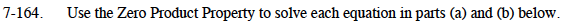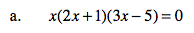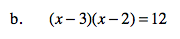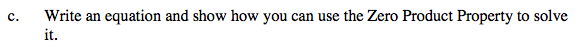Home > CCA2 > Chapter 7 > Lesson 7.2.4 > Problem7-164

7-164.
1. Use the Zero Product Property to solve each equation in parts (a) and (b) below. Homework Help ✎

1. x(2x + 1)(3x − 5) = 0

2. (x − 3)(x − 2) = 12

3. Write an equation and show how you can use the Zero Product Property to solve it.Multiply, subtract 12 from both sides, and re-factor before using the Zero Product Property.See parts (a) and (b) for examples.